# R S Aggarwal Solutions for Class 11 Maths Chapter 23 Ellipse

R S Aggarwal Solutions Class 11 Maths is among the most popular study materials. This study material helps students to learn various Maths concepts covered under the textbook. Chapter 23 of RS Aggarwal Solutions for Class 11 Maths mainly deals with topic Ellipse and terms related to it such as foci, centre, vertices, axes, eccentricity, length of latus rectum and many more. R S Aggarwal Class 11 Solutions are also helpful to practice various problems on Ellipse and help students score well in the examination.

Important points to remember:

1. For general form of ellipse:

x2/a2 + y2/b2 = 1

 Major axis Minor Axis vertices foci eccentricity latus rectum 2a 2b (±a, 0) (±c, 0) e = c/a 2b2/a

2. For general form of ellipse:

x2/b2 + y2/a2 = 1

 Major axis Minor Axis vertices foci eccentricity latus rectum 2a 2b (0, ±a) (0, ±c) e = c/a 2b2/a

## Download PDF of R S Aggarwal Solutions for Class 11 Maths Chapter 23 Ellipse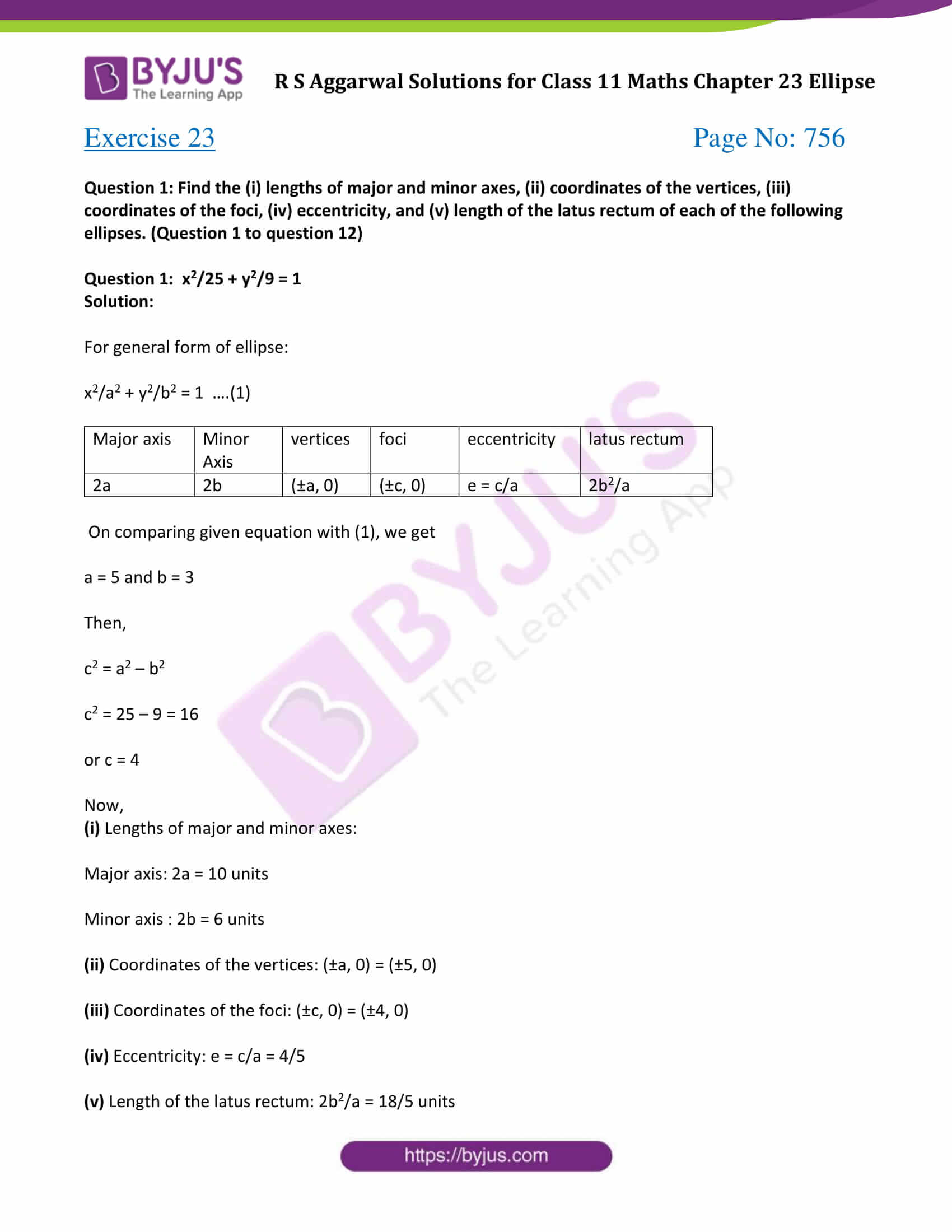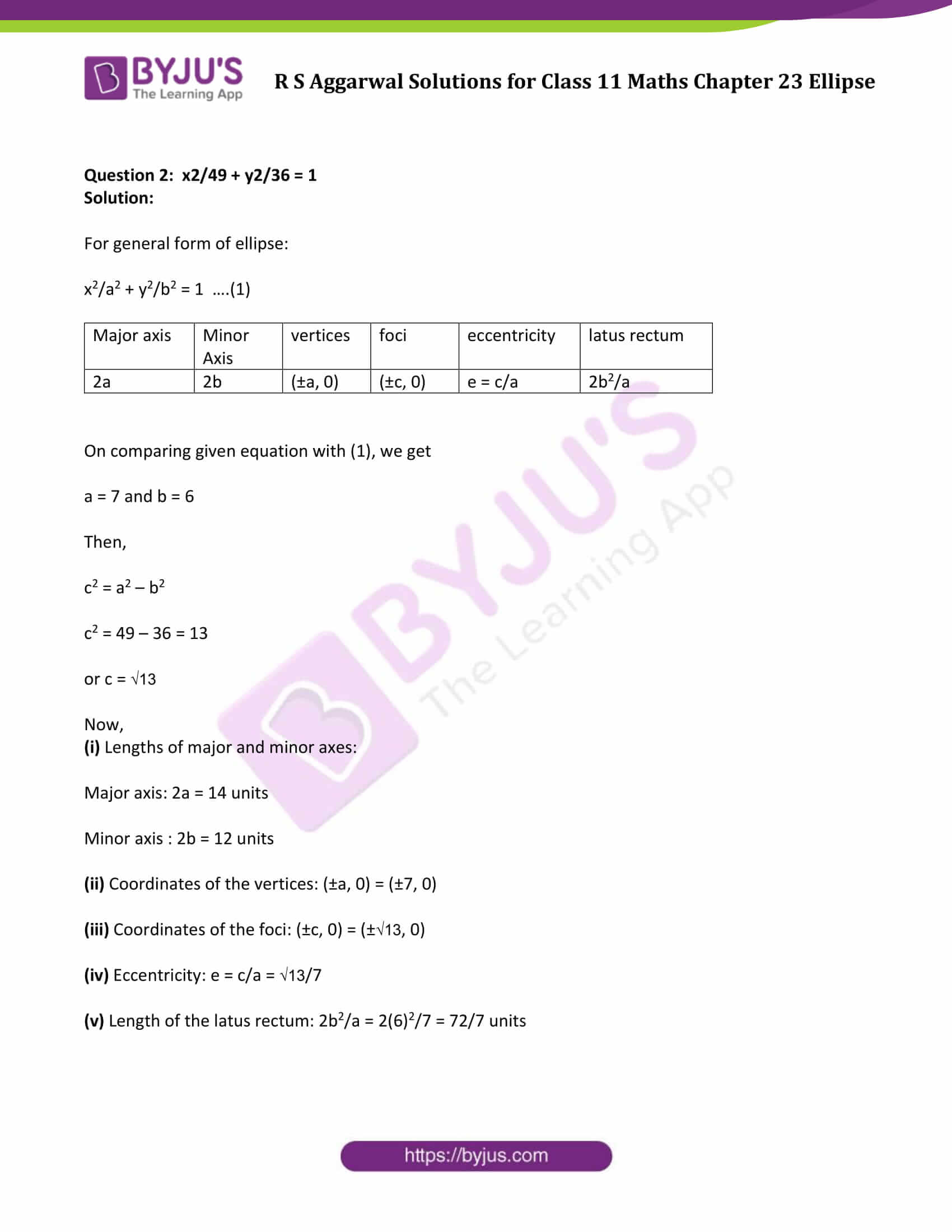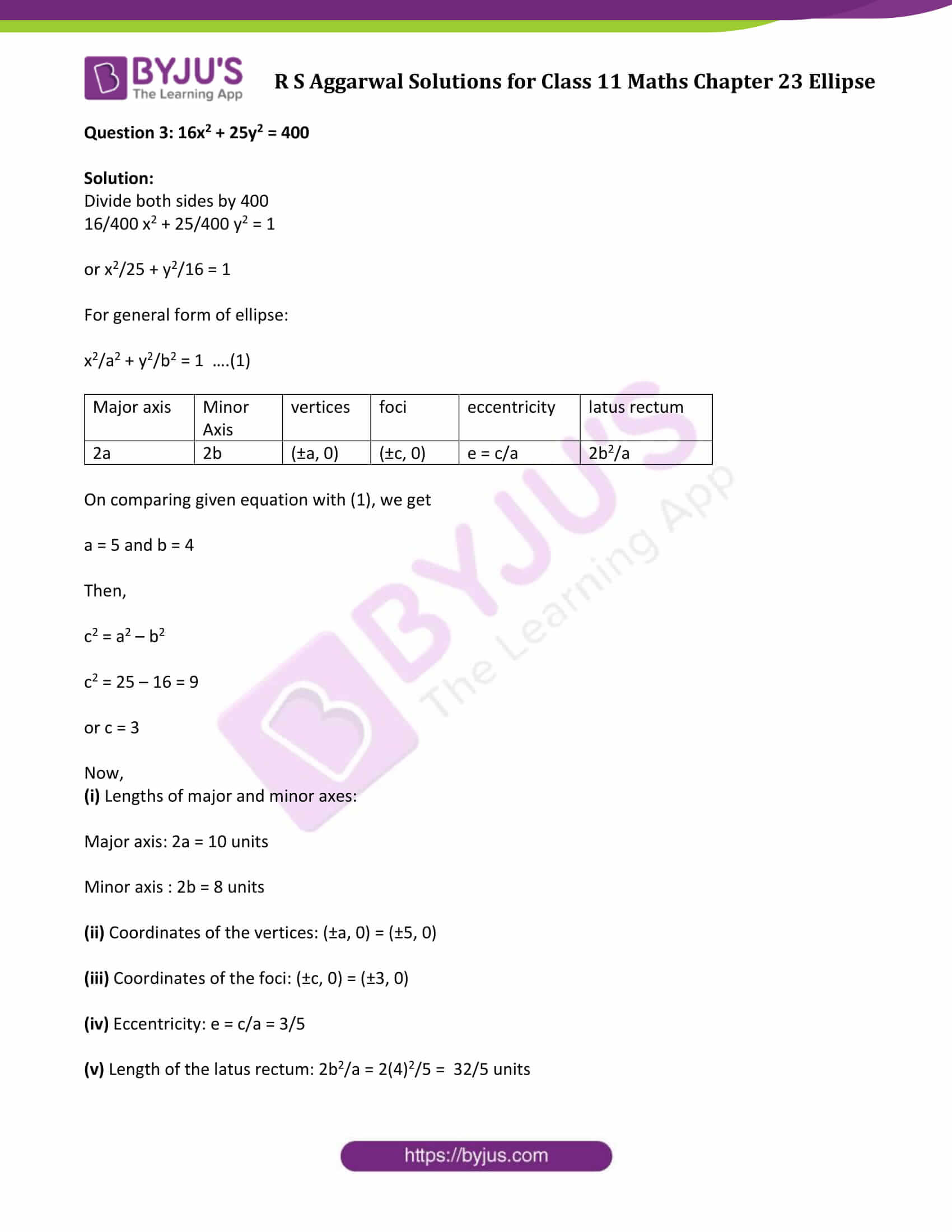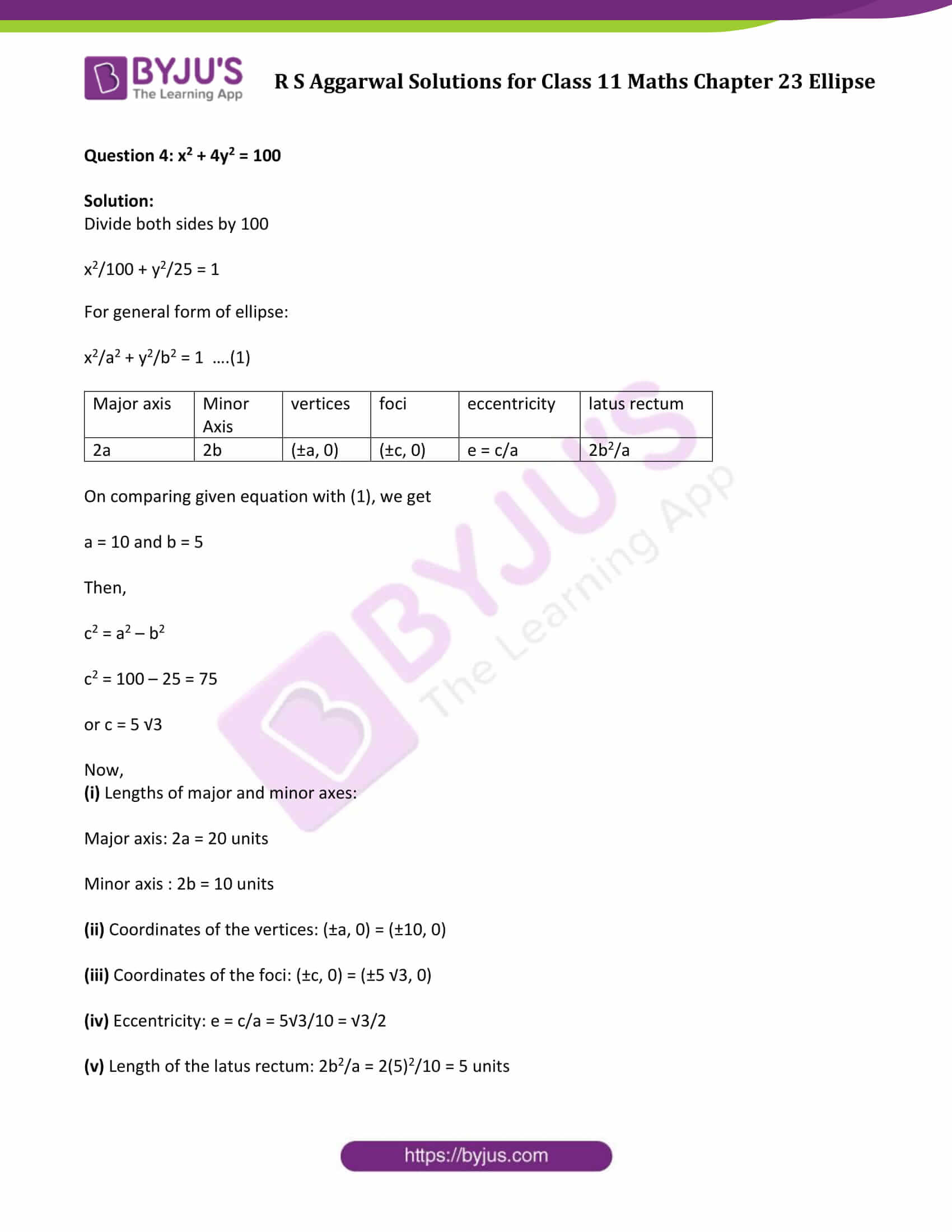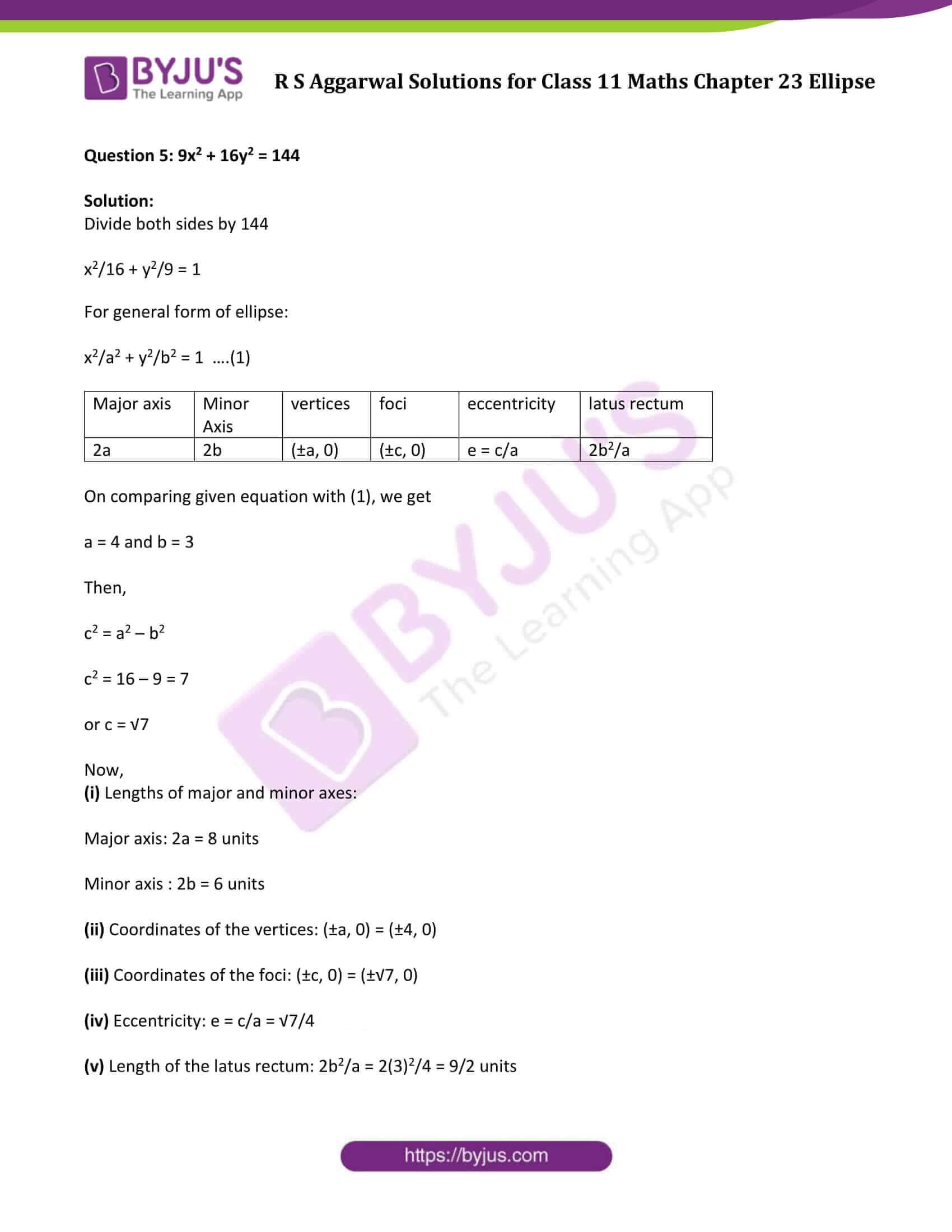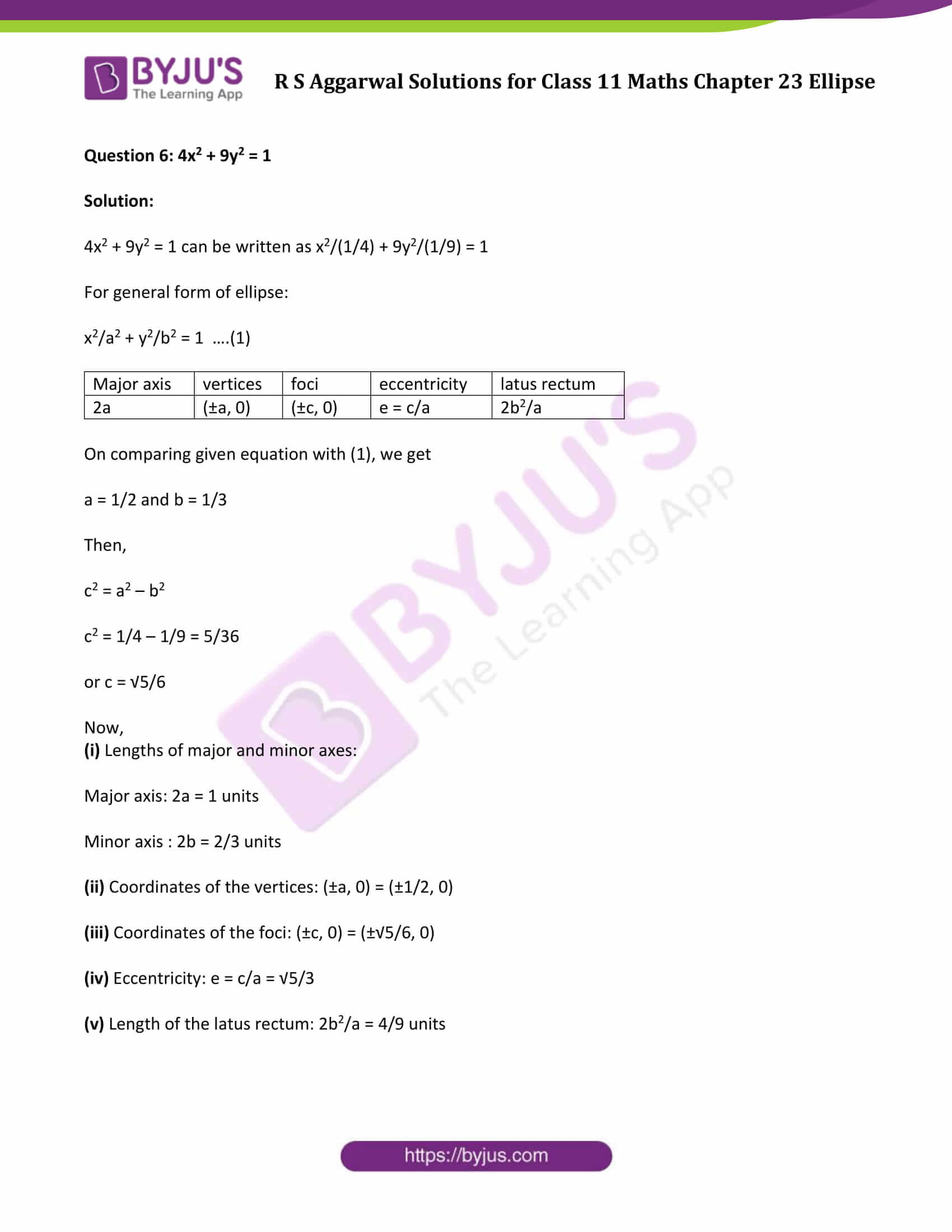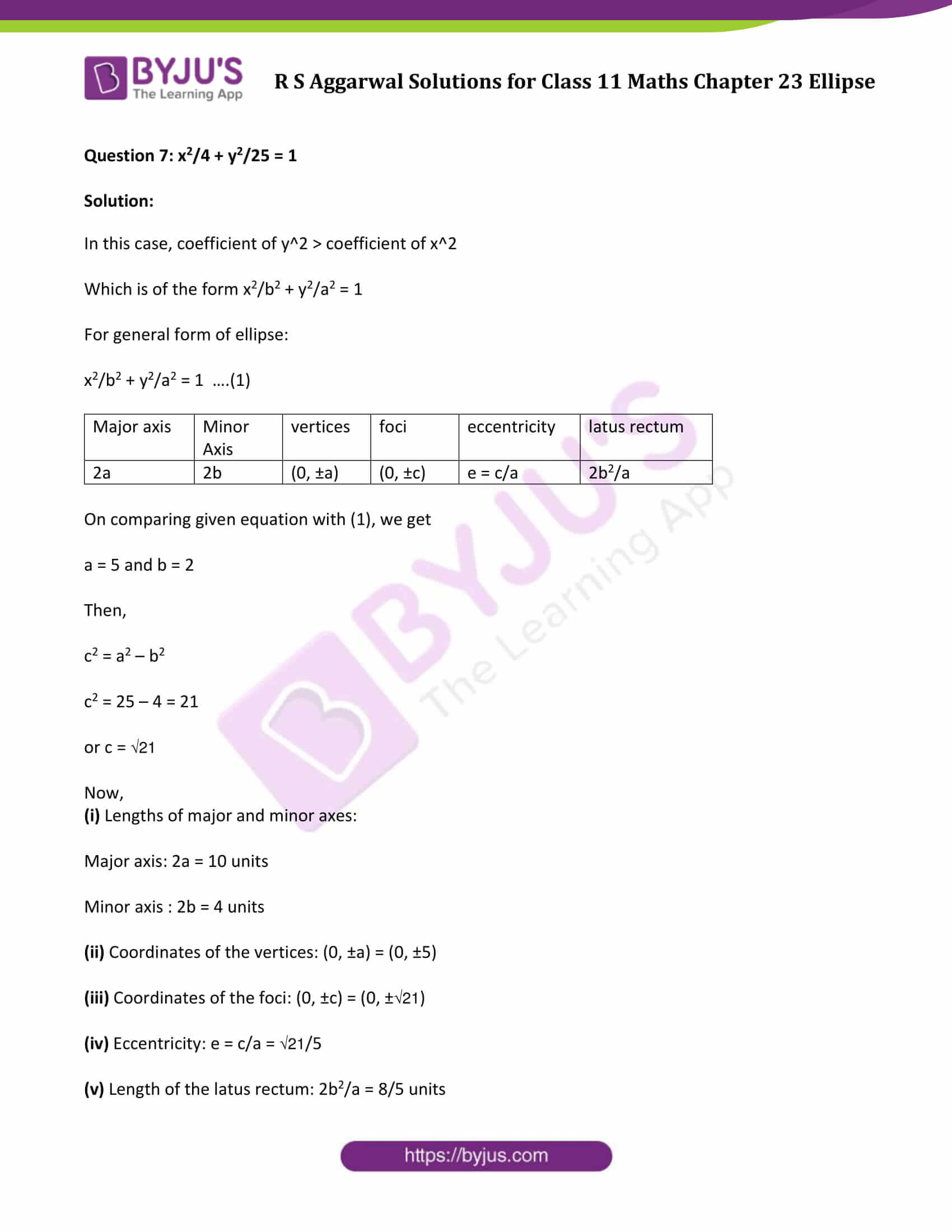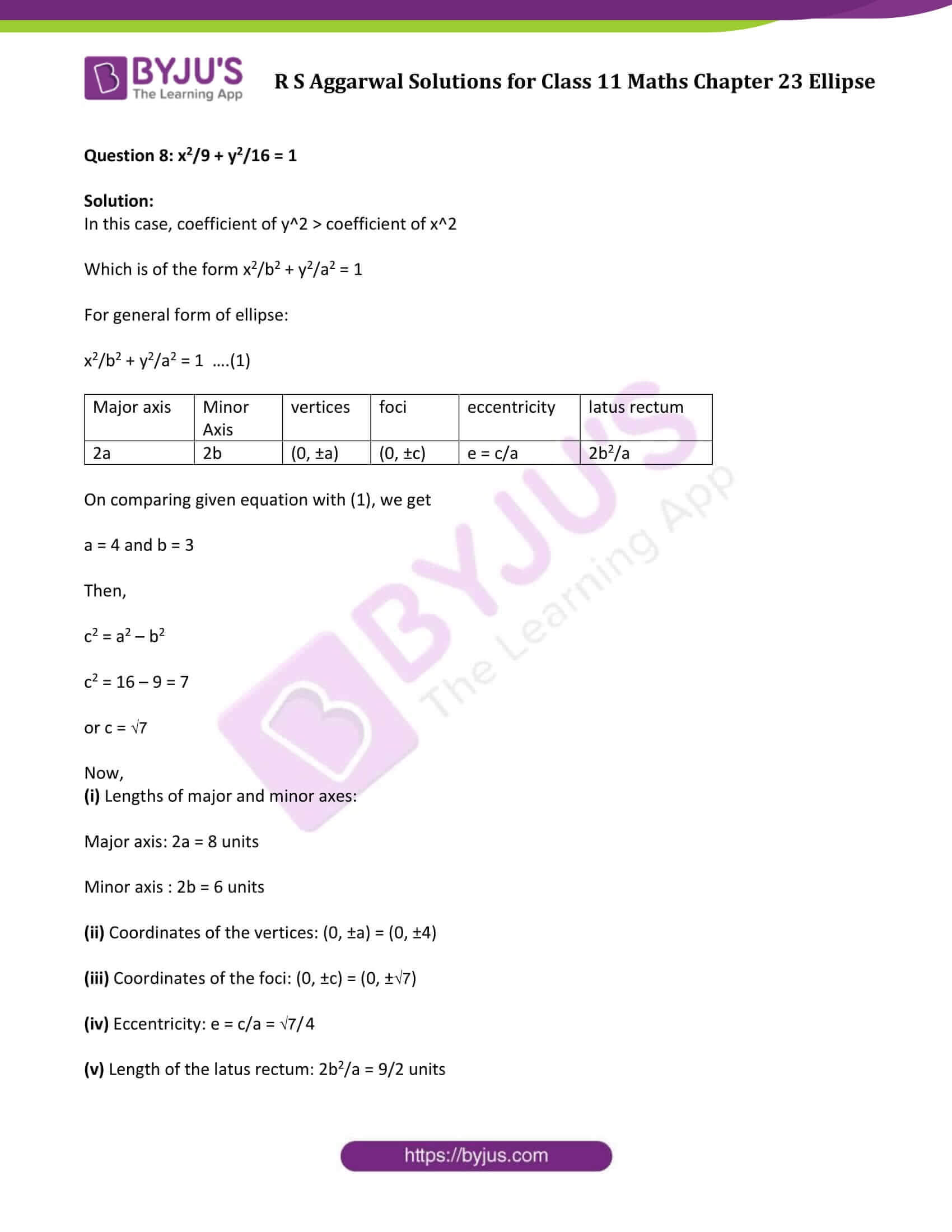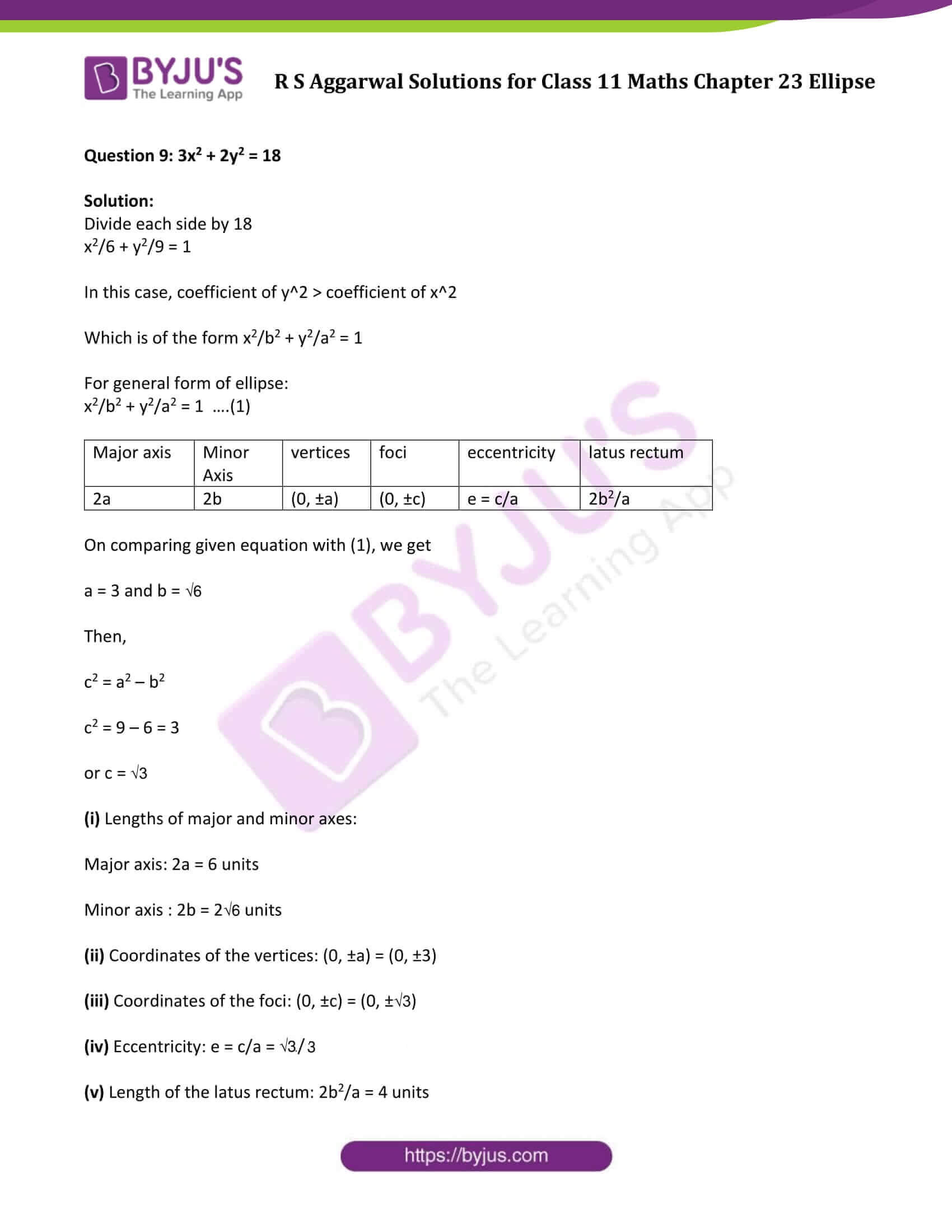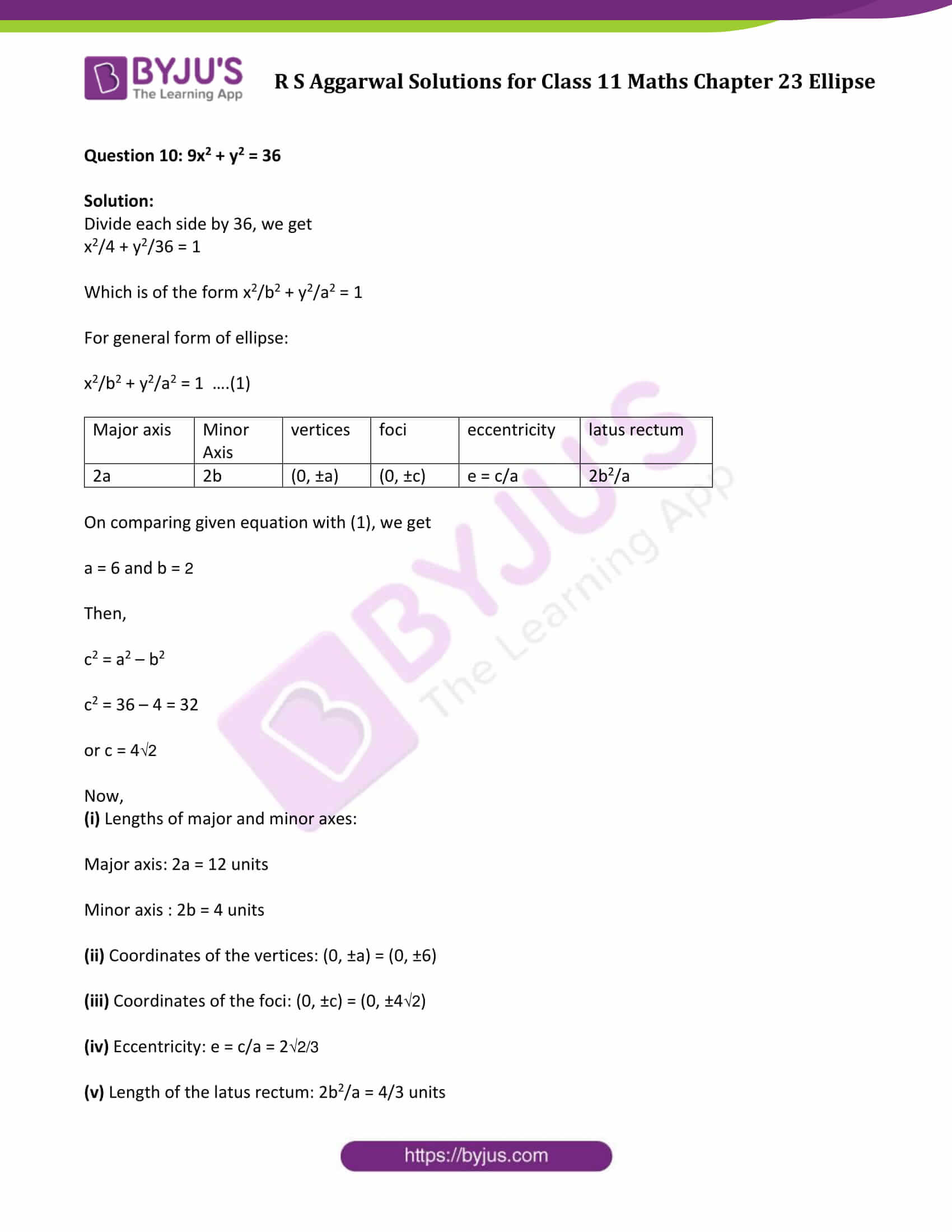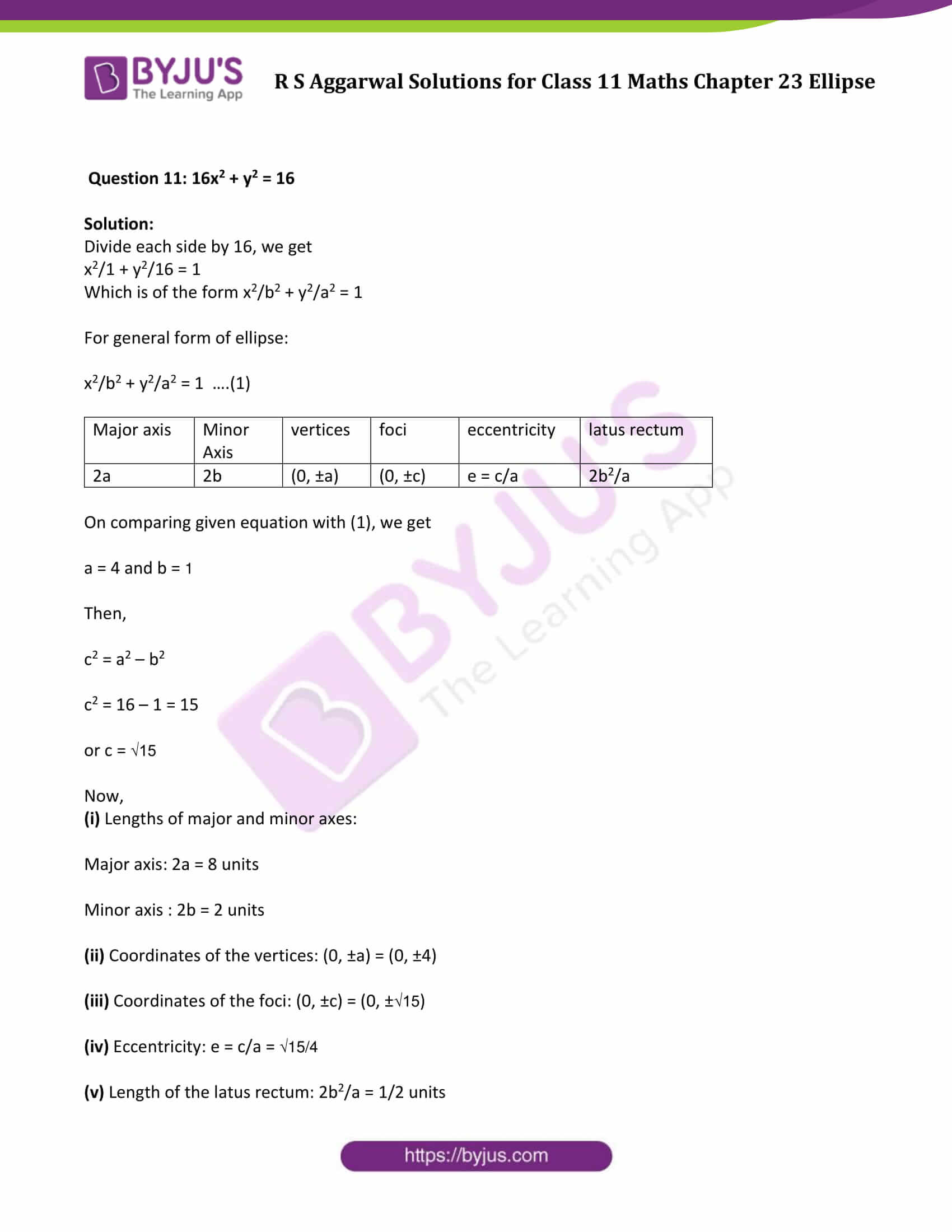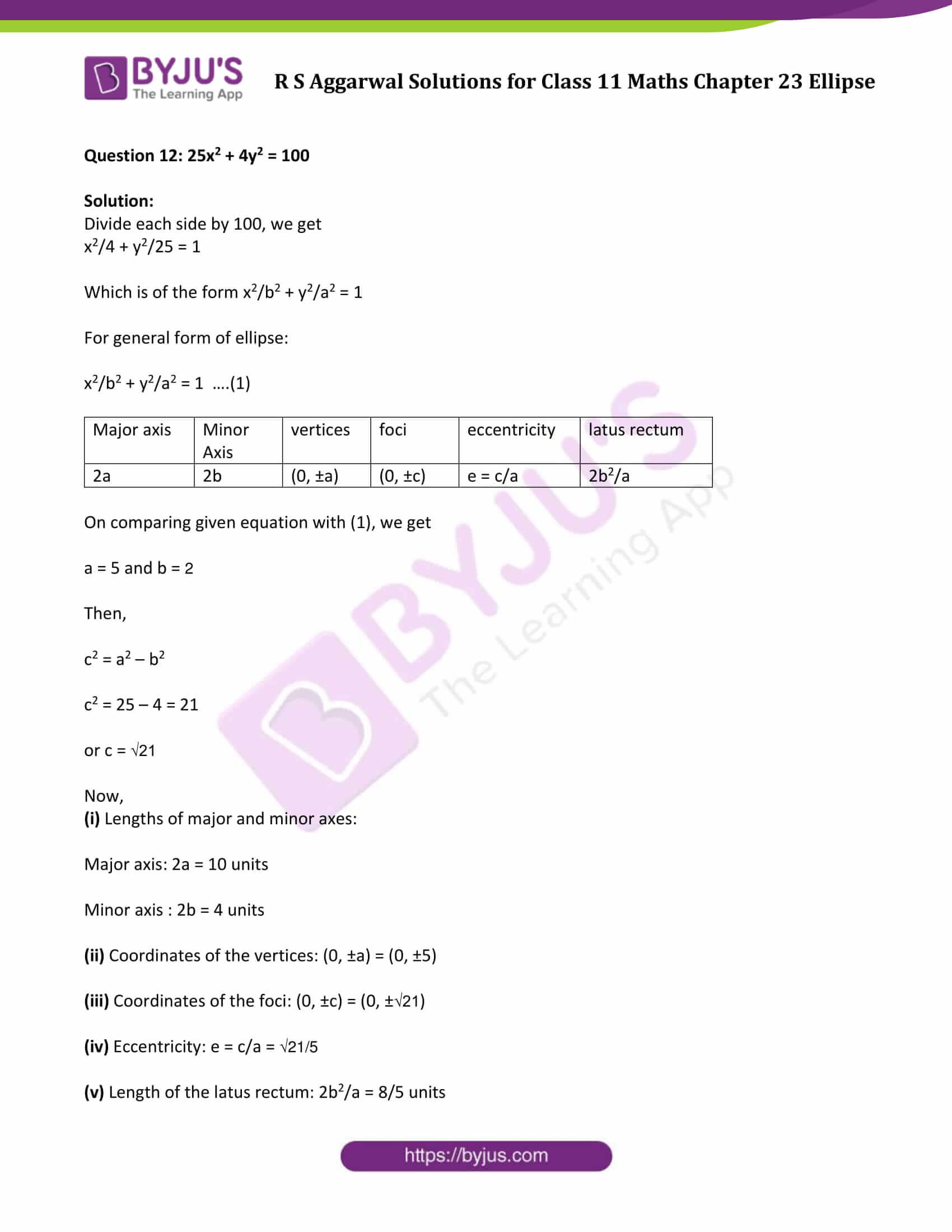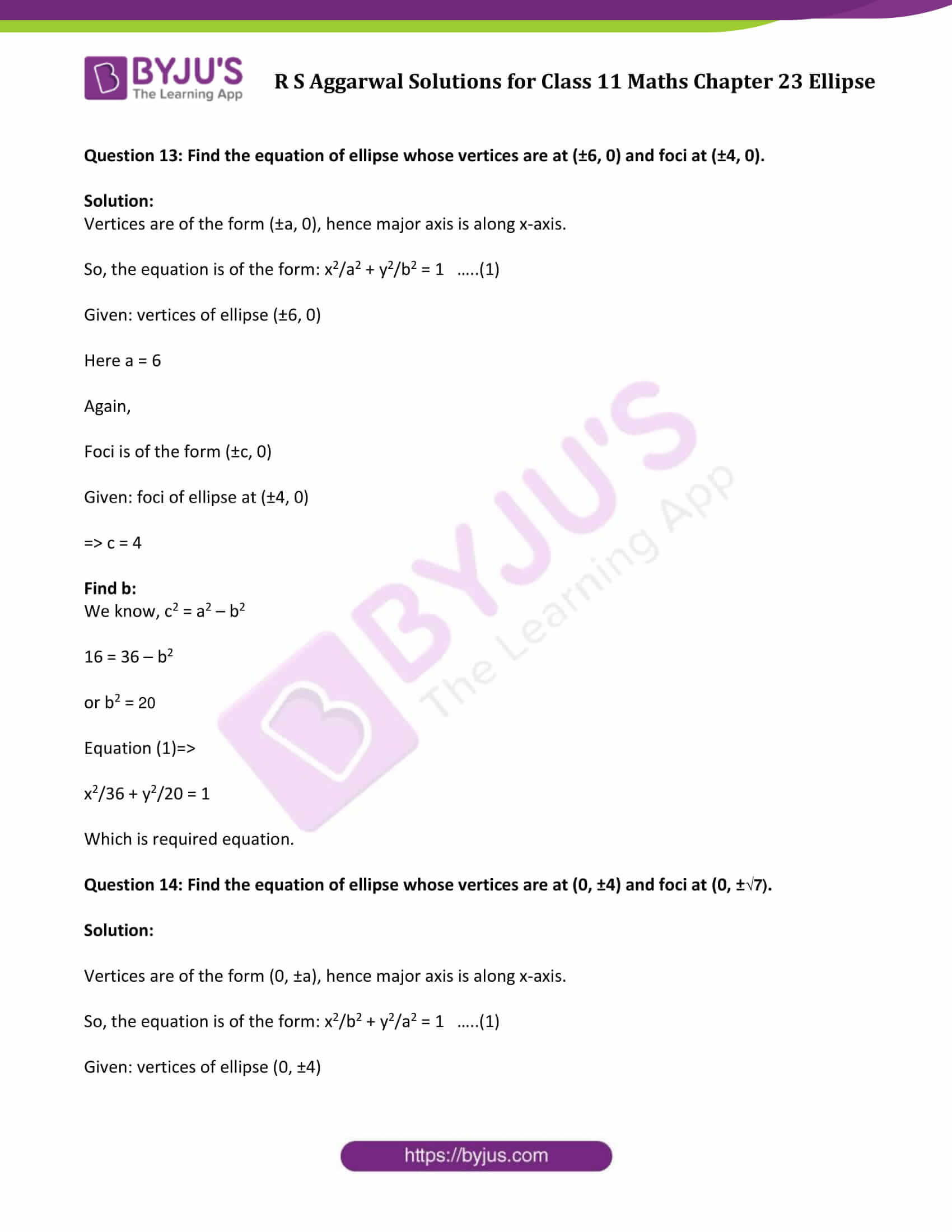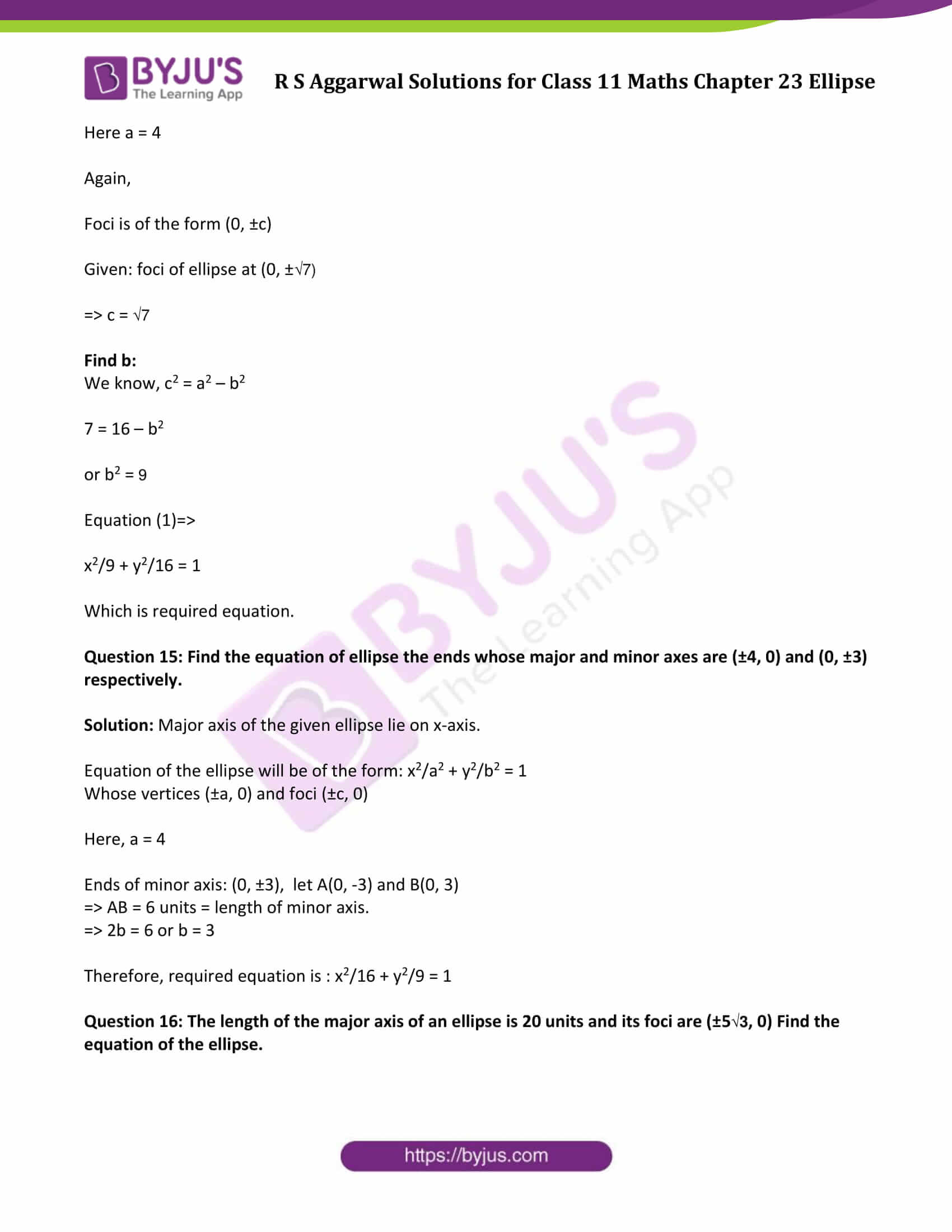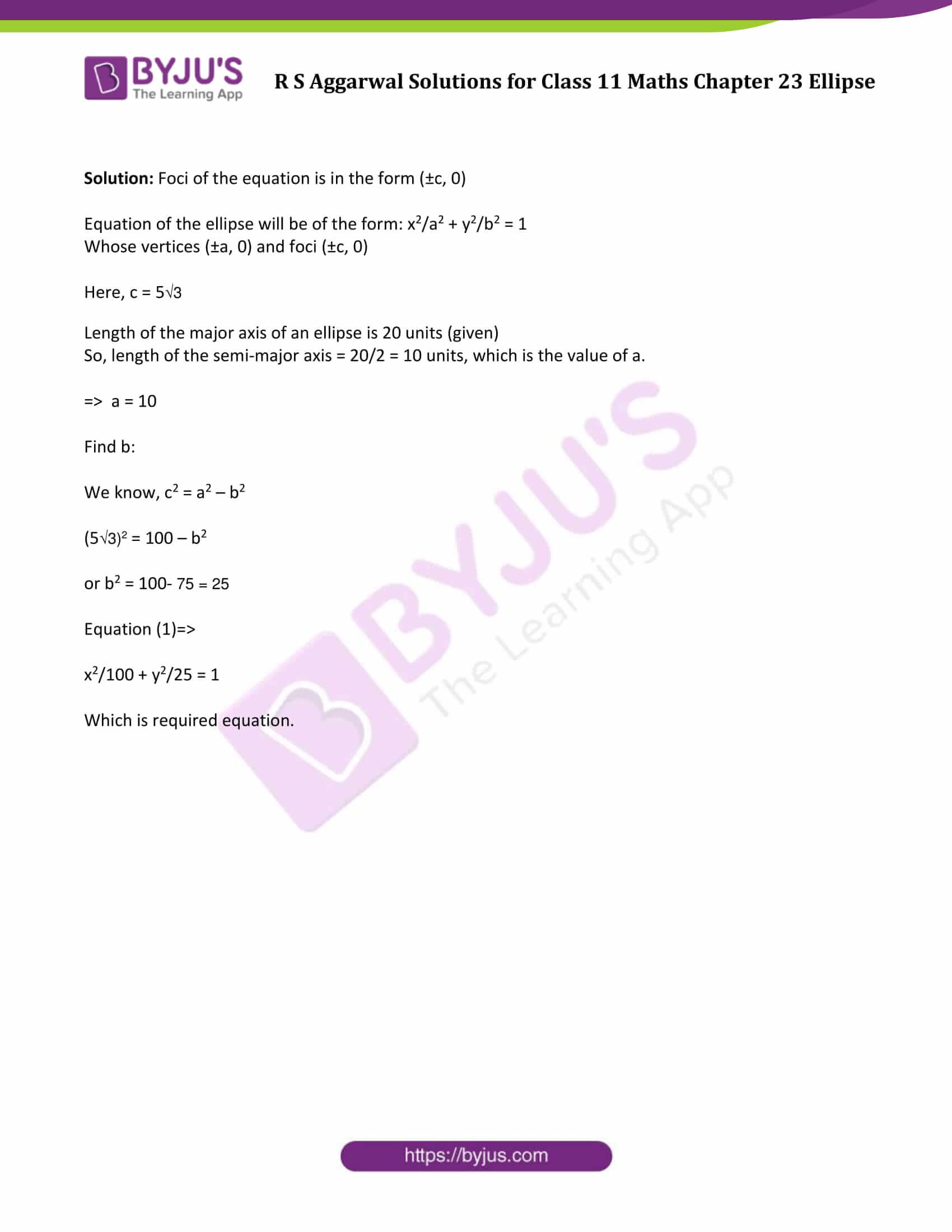### Access Answers of R S Aggarwal Solutions for Class 11 Maths Chapter 23 Ellipse

Exercise 23 Page No: 756

Question 1: Find the (i) lengths of major and minor axes, (ii) coordinates of the vertices, (iii) coordinates of the foci, (iv) eccentricity, and (v) length of the latus rectum of each of the following ellipses. (Question 1 to question 12)

Question 1: x2/25 + y2/9 = 1

Solution:

For general form of ellipse:

x2/a2 + y2/b2 = 1 ….(1)

 Major axis Minor Axis vertices foci eccentricity latus rectum 2a 2b (±a, 0) (±c, 0) e = c/a 2b2/a

On comparing given equation with (1), we get

a = 5 and b = 3

Then,

c2 = a2 – b2

c2 = 25 – 9 = 16

or c = 4

Now,

(i) Lengths of major and minor axes:

Major axis: 2a = 10 units

Minor axis : 2b = 6 units

(ii) Coordinates of the vertices: (±a, 0) = (±5, 0)

(iii) Coordinates of the foci: (±c, 0) = (±4, 0)

(iv) Eccentricity: e = c/a = 4/5

(v) Length of the latus rectum: 2b2/a = 18/5 units

Question 2: x2/49 + y2/36 = 1

Solution:

For general form of ellipse:

x2/a2 + y2/b2 = 1 ….(1)

 Major axis Minor Axis vertices foci eccentricity latus rectum 2a 2b (±a, 0) (±c, 0) e = c/a 2b2/a

On comparing given equation with (1), we get

a = 7 and b = 6

Then,

c2 = a2 – b2

c2 = 49 – 36 = 13

or c = √13

Now,

(i) Lengths of major and minor axes:

Major axis: 2a = 14 units

Minor axis : 2b = 12 units

(ii) Coordinates of the vertices: (±a, 0) = (±7, 0)

(iii) Coordinates of the foci: (±c, 0) = (±√13, 0)

(iv) Eccentricity: e = c/a = √13/7

(v) Length of the latus rectum: 2b2/a = 2(6)2/7 = 72/7 units

Question 3: 16x2 + 25y2 = 400

Solution:

Divide both sides by 400

16/400 x2 + 25/400 y2 = 1

or x2/25 + y2/16 = 1

For general form of ellipse:

x2/a2 + y2/b2 = 1 ….(1)

 Major axis Minor Axis vertices foci eccentricity latus rectum 2a 2b (±a, 0) (±c, 0) e = c/a 2b2/a

On comparing given equation with (1), we get

a = 5 and b = 4

Then,

c2 = a2 – b2

c2 = 25 – 16 = 9

or c = 3

Now,

(i) Lengths of major and minor axes:

Major axis: 2a = 10 units

Minor axis : 2b = 8 units

(ii) Coordinates of the vertices: (±a, 0) = (±5, 0)

(iii) Coordinates of the foci: (±c, 0) = (±3, 0)

(iv) Eccentricity: e = c/a = 3/5

(v) Length of the latus rectum: 2b2/a = 2(4)2/5 = 32/5 units

Question 4: x2 + 4y2 = 100

Solution:

Divide both sides by 100

x2/100 + y2/25 = 1

For general form of ellipse:

x2/a2 + y2/b2 = 1 ….(1)

 Major axis Minor Axis vertices foci eccentricity latus rectum 2a 2b (±a, 0) (±c, 0) e = c/a 2b2/a

On comparing given equation with (1), we get

a = 10 and b = 5

Then,

c2 = a2 – b2

c2 = 100 – 25 = 75

or c = 5 √3

Now,

(i) Lengths of major and minor axes:

Major axis: 2a = 20 units

Minor axis : 2b = 10 units

(ii) Coordinates of the vertices: (±a, 0) = (±10, 0)

(iii) Coordinates of the foci: (±c, 0) = (±5 √3, 0)

(iv) Eccentricity: e = c/a = 5√3/10 = √3/2

(v) Length of the latus rectum: 2b2/a = 2(5)2/10 = 5 units

Question 5: 9x2 + 16y2 = 144

Solution:

Divide both sides by 144

x2/16 + y2/9 = 1

For general form of ellipse:

x2/a2 + y2/b2 = 1 ….(1)

 Major axis Minor Axis vertices foci eccentricity latus rectum 2a 2b (±a, 0) (±c, 0) e = c/a 2b2/a

On comparing given equation with (1), we get

a = 4 and b = 3

Then,

c2 = a2 – b2

c2 = 16 – 9 = 7

or c = √7

Now,

(i) Lengths of major and minor axes:

Major axis: 2a = 8 units

Minor axis : 2b = 6 units

(ii) Coordinates of the vertices: (±a, 0) = (±4, 0)

(iii) Coordinates of the foci: (±c, 0) = (±√7, 0)

(iv) Eccentricity: e = c/a = √7/4

(v) Length of the latus rectum: 2b2/a = 2(3)2/4 = 9/2 units

Question 6: 4x2 + 9y2 = 1

Solution:

4x2 + 9y2 = 1 can be written as x2/(1/4) + 9y2/(1/9) = 1

For general form of ellipse:

x2/a2 + y2/b2 = 1 ….(1)

 Major axis vertices foci eccentricity latus rectum 2a (±a, 0) (±c, 0) e = c/a 2b2/a

On comparing given equation with (1), we get

a = 1/2 and b = 1/3

Then,

c2 = a2 – b2

c2 = 1/4 – 1/9 = 5/36

or c = √5/6

Now,

(i) Lengths of major and minor axes:

Major axis: 2a = 1 units

Minor axis : 2b = 2/3 units

(ii) Coordinates of the vertices: (±a, 0) = (±1/2, 0)

(iii) Coordinates of the foci: (±c, 0) = (±√5/6, 0)

(iv) Eccentricity: e = c/a = √5/3

(v) Length of the latus rectum: 2b2/a = 4/9 units

Question 7: x2/4 + y2/25 = 1

Solution:

In this case, coefficient of y^2 > coefficient of x^2

Which is of the form x2/b2 + y2/a2 = 1

For general form of ellipse:

x2/b2 + y2/a2 = 1 ….(1)

 Major axis Minor Axis vertices foci eccentricity latus rectum 2a 2b (0, ±a) (0, ±c) e = c/a 2b2/a

On comparing given equation with (1), we get

a = 5 and b = 2

Then,

c2 = a2 – b2

c2 = 25 – 4 = 21

or c = √21

Now,

(i) Lengths of major and minor axes:

Major axis: 2a = 10 units

Minor axis : 2b = 4 units

(ii) Coordinates of the vertices: (0, ±a) = (0, ±5)

(iii) Coordinates of the foci: (0, ±c) = (0, ±√21)

(iv) Eccentricity: e = c/a = √21/5

(v) Length of the latus rectum: 2b2/a = 8/5 units

Question 8: x2/9 + y2/16 = 1

Solution:

In this case, coefficient of y^2 > coefficient of x^2

Which is of the form x2/b2 + y2/a2 = 1

For general form of ellipse:

x2/b2 + y2/a2 = 1 ….(1)

 Major axis Minor Axis vertices foci eccentricity latus rectum 2a 2b (0, ±a) (0, ±c) e = c/a 2b2/a

On comparing given equation with (1), we get

a = 4 and b = 3

Then,

c2 = a2 – b2

c2 = 16 – 9 = 7

or c = √7

Now,

(i) Lengths of major and minor axes:

Major axis: 2a = 8 units

Minor axis : 2b = 6 units

(ii) Coordinates of the vertices: (0, ±a) = (0, ±4)

(iii) Coordinates of the foci: (0, ±c) = (0, ±√7)

(iv) Eccentricity: e = c/a = √7/4

(v) Length of the latus rectum: 2b2/a = 9/2 units

Question 9: 3x2 + 2y2 = 18

Solution:

Divide each side by 18

x2/6 + y2/9 = 1

In this case, coefficient of y^2 > coefficient of x^2

Which is of the form x2/b2 + y2/a2 = 1

For general form of ellipse:

x2/b2 + y2/a2 = 1 ….(1)

 Major axis Minor Axis vertices foci eccentricity latus rectum 2a 2b (0, ±a) (0, ±c) e = c/a 2b2/a

On comparing given equation with (1), we get

a = 3 and b = √6

Then,

c2 = a2 – b2

c2 = 9 – 6 = 3

or c = √3

(i) Lengths of major and minor axes:

Major axis: 2a = 6 units

Minor axis : 2b = 2√6 units

(ii) Coordinates of the vertices: (0, ±a) = (0, ±3)

(iii) Coordinates of the foci: (0, ±c) = (0, ±√3)

(iv) Eccentricity: e = c/a = √3/3

(v) Length of the latus rectum: 2b2/a = 4 units

Question 10: 9x2 + y2 = 36

Solution:

Divide each side by 36, we get

x2/4 + y2/36 = 1

Which is of the form x2/b2 + y2/a2 = 1

For general form of ellipse:

x2/b2 + y2/a2 = 1 ….(1)

 Major axis Minor Axis vertices foci eccentricity latus rectum 2a 2b (0, ±a) (0, ±c) e = c/a 2b2/a

On comparing given equation with (1), we get

a = 6 and b = 2

Then,

c2 = a2 – b2

c2 = 36 – 4 = 32

or c = 4√2

Now,

(i) Lengths of major and minor axes:

Major axis: 2a = 12 units

Minor axis : 2b = 4 units

(ii) Coordinates of the vertices: (0, ±a) = (0, ±6)

(iii) Coordinates of the foci: (0, ±c) = (0, ±4√2)

(iv) Eccentricity: e = c/a = 2√2/3

(v) Length of the latus rectum: 2b2/a = 4/3 units

Question 11: 16x2 + y2 = 16

Solution:

Divide each side by 16, we get

x2/1 + y2/16 = 1

Which is of the form x2/b2 + y2/a2 = 1

For general form of ellipse:

x2/b2 + y2/a2 = 1 ….(1)

 Major axis Minor Axis vertices foci eccentricity latus rectum 2a 2b (0, ±a) (0, ±c) e = c/a 2b2/a

On comparing given equation with (1), we get

a = 4 and b = 1

Then,

c2 = a2 – b2

c2 = 16 – 1 = 15

or c = √15

Now,

(i) Lengths of major and minor axes:

Major axis: 2a = 8 units

Minor axis : 2b = 2 units

(ii) Coordinates of the vertices: (0, ±a) = (0, ±4)

(iii) Coordinates of the foci: (0, ±c) = (0, ±√15)

(iv) Eccentricity: e = c/a = √15/4

(v) Length of the latus rectum: 2b2/a = 1/2 units

Question 12: 25x2 + 4y2 = 100

Solution:

Divide each side by 100, we get

x2/4 + y2/25 = 1

Which is of the form x2/b2 + y2/a2 = 1

For general form of ellipse:

x2/b2 + y2/a2 = 1 ….(1)

 Major axis Minor Axis vertices foci eccentricity latus rectum 2a 2b (0, ±a) (0, ±c) e = c/a 2b2/a

On comparing given equation with (1), we get

a = 5 and b = 2

Then,

c2 = a2 – b2

c2 = 25 – 4 = 21

or c = √21

Now,

(i) Lengths of major and minor axes:

Major axis: 2a = 10 units

Minor axis : 2b = 4 units

(ii) Coordinates of the vertices: (0, ±a) = (0, ±5)

(iii) Coordinates of the foci: (0, ±c) = (0, ±√21)

(iv) Eccentricity: e = c/a = √21/5

(v) Length of the latus rectum: 2b2/a = 8/5 units

Question 13: Find the equation of ellipse whose vertices are at (±6, 0) and foci at (±4, 0).

Solution:

Vertices are of the form (±a, 0), hence major axis is along x-axis.

So, the equation is of the form: x2/a2 + y2/b2 = 1 …..(1)

Given: vertices of ellipse (±6, 0)

Here a = 6

Again,

Foci is of the form (±c, 0)

Given: foci of ellipse at (±4, 0)

⇒ c = 4

Find b:

We know, c2 = a2 – b2

16 = 36 – b2

or b2 = 20

Equation (1)⇒

x2/36 + y2/20 = 1

Which is required equation.

Question 14: Find the equation of ellipse whose vertices are at (0, ±4) and foci at (0, ±√7).

Solution:

Vertices are of the form (0, ±a), hence major axis is along x-axis.

So, the equation is of the form: x2/b2 + y2/a2 = 1 …..(1)

Given: vertices of ellipse (0, ±4)

Here a = 4

Again,

Foci is of the form (0, ±c)

Given: foci of ellipse at (0, ±√7)

⇒ c = √7

Find b:

We know, c2 = a2 – b2

7 = 16 – b2

or b2 = 9

Equation (1)⇒

x2/9 + y2/16 = 1

Which is required equation.

Question 15: Find the equation of ellipse the ends whose major and minor axes are (±4, 0) and (0, ±3) respectively.

Solution: Major axis of the given ellipse lie on x-axis.

Equation of the ellipse will be of the form: x2/a2 + y2/b2 = 1

Whose vertices (±a, 0) and foci (±c, 0)

Here, a = 4

Ends of minor axis: (0, ±3), let A(0, -3) and B(0, 3)

⇒ AB = 6 units = length of minor axis.

⇒ 2b = 6 or b = 3

Therefore, required equation is : x2/16 + y2/9 = 1

Question 16: The length of the major axis of an ellipse is 20 units and its foci are (±5√3, 0) Find the equation of the ellipse.

Solution: Foci of the equation is in the form (±c, 0)

Equation of the ellipse will be of the form: x2/a2 + y2/b2 = 1

Whose vertices (±a, 0) and foci (±c, 0)

Here, c = 5√3

Length of the major axis of an ellipse is 20 units (given)

So, length of the semi-major axis = 20/2 = 10 units, which is the value of a.

⇒ a = 10

Find b:

We know, c2 = a2 – b2

(5√3)2 = 100 – b2

or b2 = 100- 75 = 25

Equation (1)⇒

x2/100 + y2/25 = 1

Which is required equation.

## R S Aggarwal Solutions for Chapter 23 Ellipse

R S Aggarwal Chapter 23 – Ellipse, contains the answers to all the questions listed in this chapter. Let us have a look at some of the topics that are being covered here:

• Ellipse introduction
• Standard equation of an Ellipse
• Horizontal Ellipse
• Vertical Ellipse